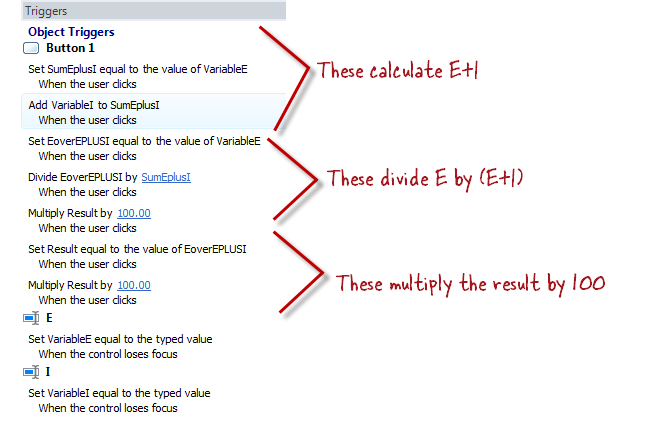Math with Variables?

Let's assume that I am performing some type of preference quiz. Based on user selections, 2 variables (let’s call them E and I) store adjusted values. So far, easy enough. Now let’s say I want to show as a percentage the strength of one over the other. In excel (or any other program supporting simple math functionality) I would use something like: 100 * (Variable X / (Variable X + Variable Y)).

My question is, can I set a new variable's value using a simple equation like this?
“Set EvIP = 100 * (Variable X / (Variable X + Variable Y)) when the user clicks…”

11 RepliesI can't remember if you can do multi-step calcs natively.  What you might be able to do each step one at a time to produce the end result.  E.g. start with a division > then a multiplication > then another division = final answer.  NOTE: those 3 steps aren't supposed to mimic your exact formula - they're just an example.  Sure, this might mean introducing another couple of variables but logically (and I'm not in front of SL right now) I see no reason for it not to work.Hi Owen! You can certainly do that. You'll need triggers for each operation within the equation, though. Attached at the bottom of this post is an example file showing your equation of 100*(E/(E+I)) ... go ahead and preview it in Storyline and see if this mimics what you had in mind.

There might be other ways to do this, but to me (and my non-math-brain) it made the most sense to create a variable for not only E and I, but also the sum of E+I and the final result. Then I used those variables to work my way through the equation. Here's the logic I used in my triggers:If you're down with using JavaScript, you'd be able to call this whenever you need to run the calculation.

For this one:

Set EvIP = 100 * (Variable X / (Variable X + Variable Y))

Make a JavaScript trigger like this:

var player=GetPlayer();

var vX=player.GetVar("VariableX");

var vY=player.GetVar("VariableY");

player.SetVar("EvIP",(vX/(vX+vY))*100);

Now you can just copy this trigger whenever and it'll set EvIP to the calculated value.Hi Owen - here's a quick example. In the .story file attached below, I inserted a character and created a couple of states for him (the states just show the character in a different expression/pose). Then I added some triggers which change the character's state based on the outcome of the calculation.  Here's a screenr that shows how:  https://jeanette.viewscreencasts.com/aa920f4367005c834623a473f848c643